﻿ 基于CFD数据库及NMF的流场重构成像方法

# 基于CFD数据库及NMF的流场重构成像方法Flow Field Reconstruction Tomography Method Based on CFD Database and NMF

Abstract: This paper proposes a new process tomography (PT) method based on computational fluid dynamics (CFD) and non-negative matrix factorization (NMF) for reconstructing flow fields. The CFD sample database is established by CFD simulation, and the sample base matrix is extracted from the sample database by using NMF. Then, based on the basis matrix, the sample matrix can be obtained by inversely solving from a small amount of sensor measurement data, thereby reducing the sampling points required for reconstruction and realizing rapid dimensional reconstruction of the flow field. It can be seen from the reconstruction results that the method can accurately reconstruct the flow field, especially the process parameters without large order of magnitude difference; compared with other multi-phase flow detection methods, the method avoids the complex calculations of sensitive field in the multi-phase flow detection process. Meanwhile, compared to simple CFD simulation methods and interpolation methods, the time required for reconstruction is greatly reduced.

1. 引言

2. 数学模型

2.1. 重构方法数学描述

$V=AY$ (1)

$Y$ ——实际过程参数矩阵(如：流速，体积分数等)，维度为 $m×1$

$A$ ——已知变换矩阵，维度为 $x×m$

$V$ ——测量所得的过程参数矩阵，维度为 $x×1$

${Y}_{R}=W\alpha$ (2)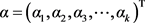${Y}_{R}=\underset{i=1}{\overset{k}{\sum }}{\alpha }_{i}{W}_{i}$ (3)

${Y}_{R}$ ——重构过程参数矩阵，维度为 $m×1$

$W$ ——基矩阵，维度为 $m×k$

$\alpha$ ——重构系数矩阵，维度为 $k×1$ ( $k\ll m$ )；

${\alpha }_{i}$ ——第 ${i}^{th}$ 节点基矩阵线性组合系数。

${Z}_{m×n}={W}_{m×k}{H}_{k×n}+{D}_{m×n}$ (4)

$Z$ ——流场过程参数样本矩阵(包含n种不同工况)，维度为 $m×n$

$W$ ——基矩阵，维度为 $m×k$

$H$ ——分解权重矩阵，维度为 $k×n$

$D$ ——分解误差矩阵，维度为 $m×n$

$Z$ 表示为低秩矩阵 $W$$H$ 的乘积加上 $D$ ，这种数学变换有助于减小问题的维数并获得主要的信息。

$V\approx AW\alpha$ (5)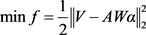(6)

${e}_{R}=\frac{{‖Y-{Y}_{R}‖}_{1}}{{‖{Y}_{R}‖}_{1}}$ (7)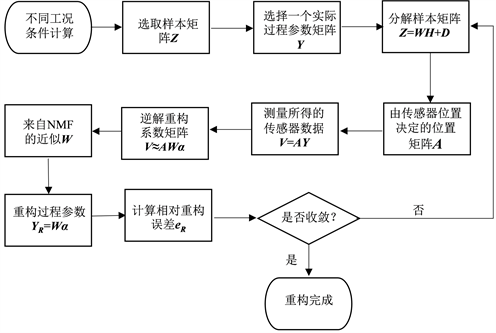Figure 1. MNR schematic diagram

2.2. 非负矩阵分解(NMF)

${Z}_{m×n}={W}_{m×k}{H}_{k×n}+{D}_{m×n}$ (8)

${Z}_{m×n}\approx {W}_{m×k}{H}_{k×n}={\stackrel{^}{Z}}_{m×n}$ (9)

where ${W}_{m×k}\ge 0$${H}_{k×n}\ge 0$

${‖B-C‖}^{2}={\underset{i,j}{\sum }\left({B}_{i,j}-{C}_{i,j}\right)}^{2}$ (10)

Kullback-Leibler散度法：

$F\left(B‖C\right)=\underset{i,j}{\sum }\left({B}_{i,j}\mathrm{log}\frac{{B}_{i,j}}{{C}_{i,j}}-{B}_{i,j}+{C}_{i,j}\right)\ge 0$ ，(当且仅当 $B=C$$F=0$ ) (12)

$\mathrm{min}{‖Z-WH‖}^{2},\text{ }s.t.W\ge 0,H\ge 0$ (11)

$\mathrm{min}F\left(Z‖WH\right),\text{ }s.t.W\ge 0,H\ge 0$ (12)

3. CFD模型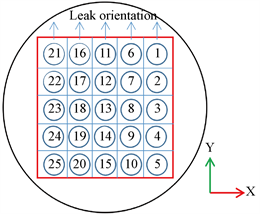(a)(b)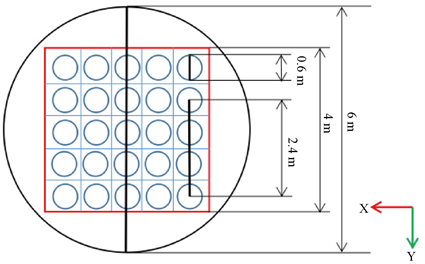(c)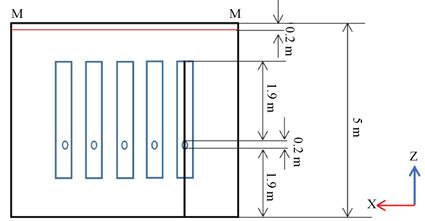(d)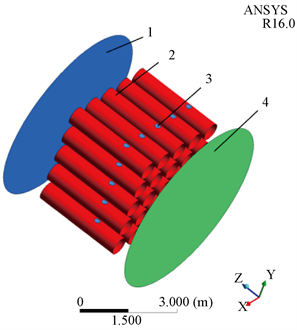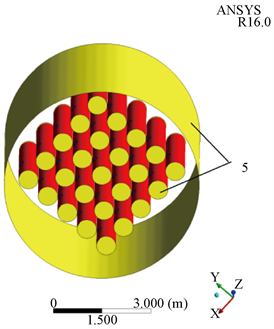1-Coolant outlet; 2-Heating rod; 3-Leaking material inlet; 4-Coolant inlet; 5-Adiabatic wall(e)

Figure 2. Model size and boundary type. (a) Multi-tube space flow model; (b) Heating rod name; (c) Top view of the geometric model; (d) Front viewof the geometric model; (e) Model in ICEM

4. 重构结果

4.1. 体积分数重构结果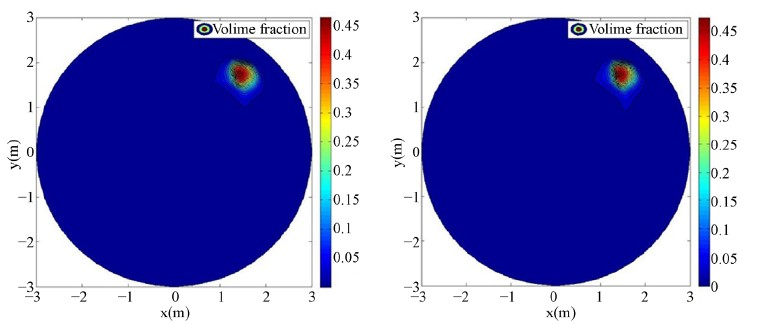(a) (b)

Figure 3. Volume fraction reconstruction result when the leakage velocity of 1 is 0.6 m/s. (a) Actual result; (b) Reconstruction result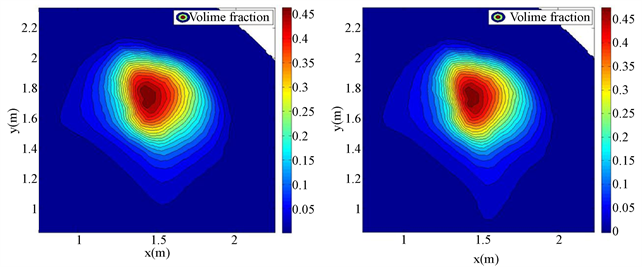(a) (b)

Figure 4. Partial enlargement of the volume fraction reconstruction result when the leakagevelocity is 0.6 m/s. (a) Actual result; (b) Reconstruction result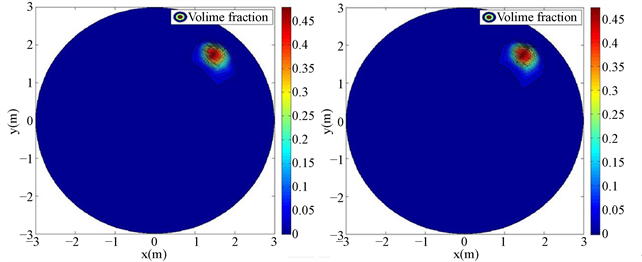(a) 实际结果 (Actual result) (b) 重构结果 Reconstruction result

Figure 5. Volume fraction reconstruction results when the leakage velocity of 1 is 0.7 m/s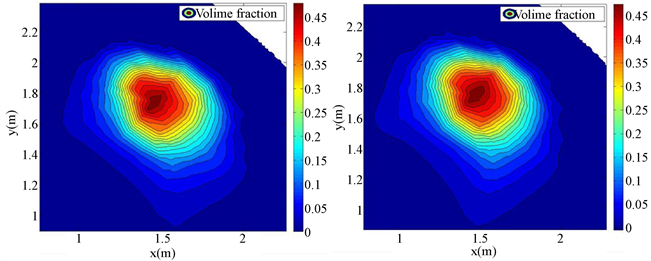(a) 实际结果 (Actual result) (b) 重构结果 Reconstruction result

Figure 6. Partial enlargement of the volume fraction reconstruction result when the leakagevelocity is 0.7 m/s

4.2. 速度重构结果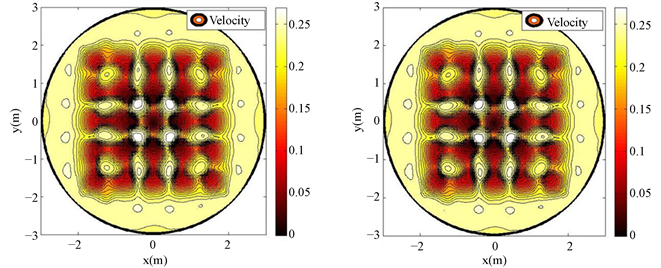(a) 实际结果 (Actual result) (b) 重构结果 Reconstruction result

Figure 7. Reconstruction result of velocity

5. 讨论

5.1. 基矩阵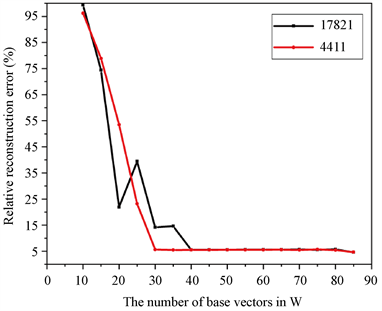Figure 8. Reconstruction result of different basis matrices

5.2. 采样率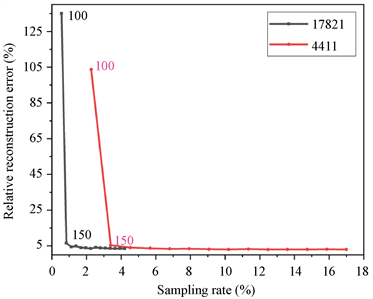Figure 9. Relative reconstruction error corresponding to different sampling rates

5.3. 泄露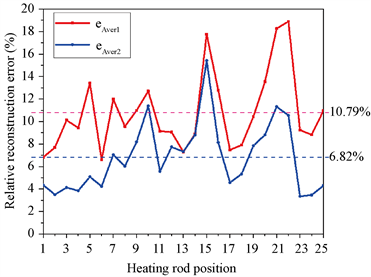Figure 10. Average relative reconstruction error of 25 heating rods

6. 结论

1. 基于有限的过程参数测量数据，所提出的方法可用于重构复杂机械结构周围的流场。不同泄露情况下，都可以较为准确地重构流场，尤其是对于类似流场速度这类不存在较大数量级差异的过程参数，可以精确地重构流场。

2. 相对重构误差主要受基矩阵、采样率以及过程参数数量级的影响。在选取合适的基矩阵的前提下，采样率越低重构误差越大并且过程参数数量级的差异是误差的主要来源。

3. 在实际情况中，所提出的重构方法避免了多相流检测过程中复杂敏感场的计算，而且相较于CFD模拟方法和插值方法，重构所需的时间大幅缩减。

 邢天阳. 多相流检测研究进展[J]. 科技资讯, 2017, 15(27): 113-114.

 马平, 周晓宁, 田沛. 过程层析成像技术的发展及应用[J]. 化工自动化及仪表, 2009, 36(1): 1-5.

 史志才, 等. 电容层析成象技术在两相流流型辨识中的应用[J]. 自动化仪表, 2000(8): 17-19.

 徐苓安. 多相流检测技术进展: 关于过程层析成像技术的评述[M]. 北京: 石油工业出版社, 1996: 7-11.

 Tallon, S.J. and Davies, C.E. (2000) The Effect of Pipeline Location on Acoustic Measurement of Gas Solid Pipeline Flow. Flow Measurement and Instrumentation, 11, 165-169.
https://doi.org/10.1016/S0955-5986(00)00015-7

 董峰, 等. 过程层析成像技术综述[J]. 仪器仪表用户, 2001, 8(1): 6-11.

 杨莉松, 王桂英, 徐至展. 光学层析成像技术的发展现状[J]. 激光与光电子学进展, 1997, 34(8): 5-7.

 Uchiyama, H., Nakajima, M. and Yuta, S. (1985) Measurement of Flame Temperature Distribution by IR Emission Computed Tomography. Applied Optics, 24, 4111-4116.
https://doi.org/10.1364/AO.24.004111

 Hertzhm, F. (1988) Emission Tomography of Flame Radicals. Optics Letters, 13, 351-353.
https://doi.org/10.1364/OL.13.000351

 阎春生, 曾楠. 光学层析成像技术的研究动态[J]. 激光杂志, 2001, 22(5): 5-7.

 York, T.A. (2001) Status of Electrical Tomography Industrial Applications. Journal of Electronic Im-aging, 10, 608-619.
https://doi.org/10.1117/1.1377308

 Holder, D. (2004) Techniques in Electrical Impedance Tomography (Series in Medical Physics and Biomedical Engineering).

 Dickin, F. and Wan, M. (1996) Electrical Resistance Tomography for Process Applications. Measurement Science and Technology, 7, 247-260.
https://doi.org/10.1088/0957-0233/7/3/005

 Huang, S.M., Plaskowski, A.B., Xie, C.G. and Beck, M.S. (1988) Capacitance-Based Tomographic Flow Imaging System. Electronics Letters, 24, 418-419.
https://doi.org/10.1049/el:19880283

 郝建娜. 电磁层析成像与检测技术逆问题的研究[D]: [博士学位论文]. 天津: 天津大学, 2013.

 Qin, L., Liu, S. and Kang, Y. (2018) Wind Velocity Distribution Reconstruction Us-ing CFD Database with Tucker Decomposition and Sensor Measurement. Energy, 167, 1236-1250.
https://doi.org/10.1016/j.energy.2018.11.013

 Lee, D.D. and Seung, H.S. (1999) Learning the Parts of Objects by Non-Negative Matrix Factorization. Nature, 401, 788-791.
https://doi.org/10.1038/44565

 Lee, D.D. and Seung, H.S. (2001) Algorithms for Non-Negative Matrix Factorization. In: Advances in Neural Information Processing Systems 13: Proceedings of the 2000 Conference, MIT Press, Cambridge, 556-562.

Top